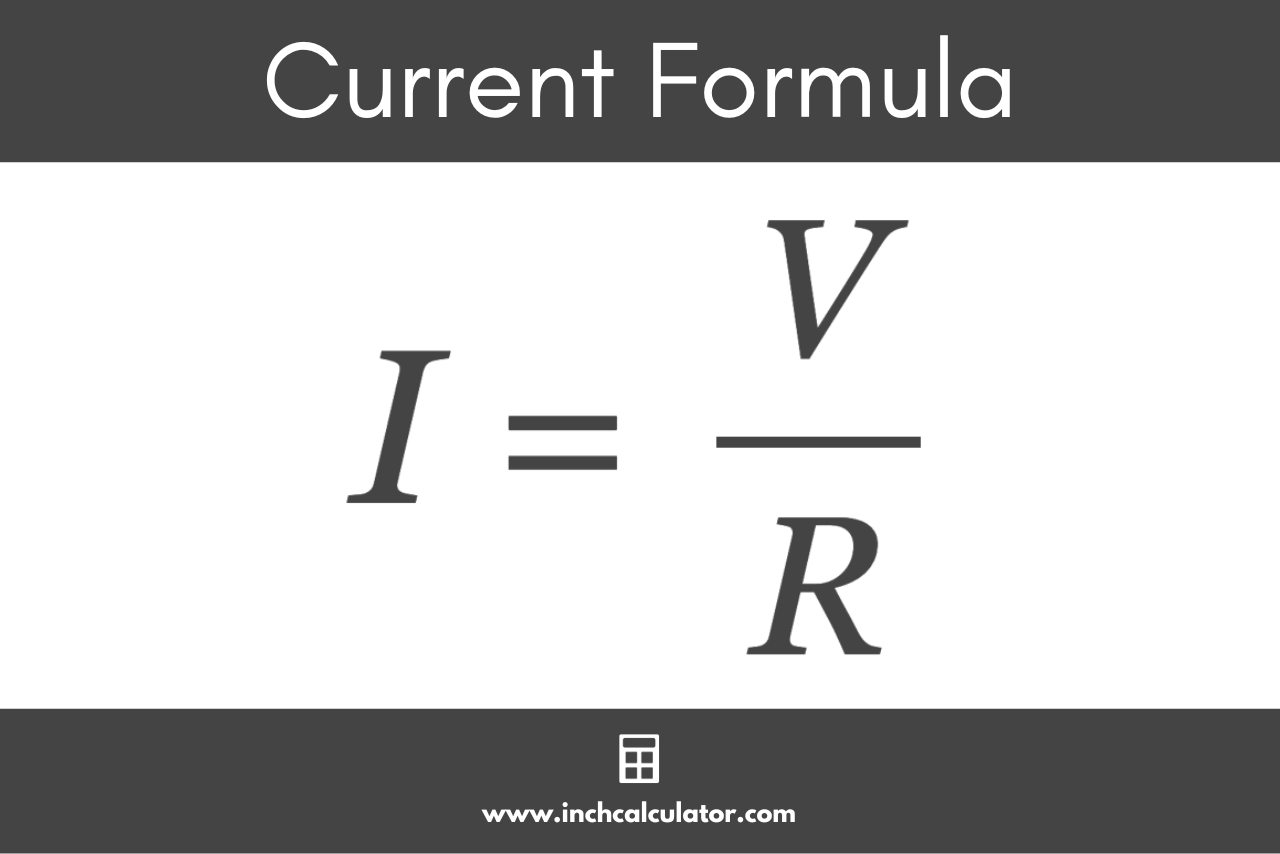# Current Calculator – Calculate Amps

Use the current calculator below to calculate amps given the voltage, power, or resistance.

## Calculation Formulas:

Learn how we calculated this below

## How to Calculate Electric Current

In an electrical circuit, current is a measure of the flow of charged particles moving through a conductor. Current is measured in amperes (usually referred to as amps).

You can calculate the current flowing through a conductor using Ohm’s Law, which states that the current through a circuit element is directly proportional to the potential difference applied to it and inversely proportional to the resistance.

### Current Formula

The Ohm’s Law formula to calculate current is I = V/R, where I is the current through the conductor in amps, V is the potential difference across the conductor in volts, and R is the resistance of the conductor in ohms.

I = V / R

Thus, the current flowing through the conductor I is equal to the voltage across the conductor V divided by the resistance of the conductor R.## How to Calculate Current Using Watts

You can also calculate electric current in amps if you know the power drawn from the circuit using the Watt’s Law power formula. The power formula states that the current in amps is equal to the power in watts divided by the voltage.

I(A) = P(W) / V(V)

The current I in amps is equal to the power P in watts divided by the voltage V.

This is the formula that is used to convert watts to amps or to convert volts to amps.

### AC Circuits Current Formula

For a single-phase AC circuit given a power factor, you can calculate the current using the following formula.

I(A) = P(W) / V(V) × PF

The current I in amps is equal to the power P in watts divided by the voltage V times the power factor PF.

You can calculate the power factor using a power factor calculator if needed.

### Three-Phase AC Current Formula

To calculate the current for three-phase AC circuits, you need to use a different formula to account for the three phases.

#### Line to Line Voltage Formula

If you know the line to line voltage in a three-phase AC circuit, you can use the following formula to calculate the current:

I(A) = P(W) / VL-L(V) × PF × √3

The current I in amps is equal to the power P in watts divided by the line to line voltage V in volts times the power factor PF times the square root of 3.

This formula calculates the current draw for a single pair of wires in three-phase systems. You’ll need to multiply the result by 3 to calculate the total current for all three pairs.

#### Line to Neutral Voltage Formula

If you know the line to neutral voltage in a three-phase AC circuit, you can use the following formula to calculate the current:

I(A) = P(W) / VL-N(V) × PF × 3

The current I in amps is equal to the power P in watts divided by the product of the line to neutral voltage V in volts, the power factor PF, and 3.

This formula calculates the total current for all three wires in a three-phase system. You’ll need to divide the result by 3 to find the current for a single wire in the circuit.

## References

1. Brandon Mitchell, Robert Ekey, Roy McCullough, and William Reitz, A Fan-tastic Quantitative Exploration of Ohm’s Law, https://aapt.scitation.org/doi/full/10.1119/1.5021431
2. National Institute of Standards and Technology, Ampere: The Present, https://www.nist.gov/si-redefinition/ampere-present
3. Miller, C., NFPA's Electrical References, National Fire Protection Association, 2004, Jones & Bartlett Learning, 67-75. https://www.google.com/books/edition/NFPA_s_Electrical_References/raUyIi7i-asC
4. Miller, C., Ugly’s Electrical References, 2020 Edition, 2020, Jones & Bartlett Learning, 16-23. https://books.google.com/books?id=1kS8DwAAQBAJ